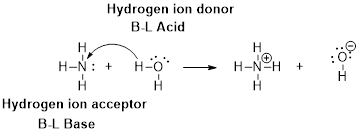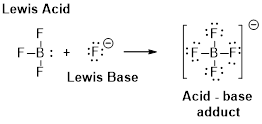Chapter 16, Problem 5PS

Chapter
Section
Textbook Problem

Write balanced equations showing how the hydrogen oxalate ion, HC2O4˗, can be both a Bronsted acid and a Bronsted base.

Interpretation Introduction

Interpretation:

The balanced equation has to be written for hydrogen oxalate (HC2O4) and the explanation has to be given for the hydrogen oxalate (HC2O4) can act as both conjugated acid and conjugated base.

Concept introduction:

Arrhenius Acids and Bases

Acid release hydrogen ion in water

Base release hydroxide ions in water.

HCl(aq) H+(aq) + Cl-(aq).........AcidNaOH(aq) Na+(aq)+ OH-(aq)...........Base

An acid is a substance that produces hydronium ions, H3O+ when dissolved in water.

Bronsted –Lowry definitions:

A Bronsted –Lowry acid is a proton donor, it donates a hydrogen ion, H+

A Bronsted-Lowry base is a proton acceptor, it accepts a hydrogen ion H+.Lewis definition:

A Lewis acid is a substance that can accept and share an electron pair.

A Lewis base is a substance that can donate and share an electron pair.Bronsted –Lowry conjugate acid-Base pairs:

When an acid is dissolved in water, the acid (HA) donates a proton to water to form a new acid (conjugate acid) and a new base (conjugate base).Explanation

Oxalate ion can act as both acid and base by lose and gain of proton. It is an amphoteric.

Act as acid:

H2C2O4- act as an acid by donation of proton to water.

The chemical reaction is as follows.

H2C2O4-(aq) + H2O  C2O42-(aq) + H3O+(aq)acid                 Base

Act as base:

H2C2O4- can act as base by accepting one proton from water.

The chemical reaction is as follows.

H2C2O4- + H2  H2C2O4+ OH-Base          Acid

Thus, oxalate ion act as both conjugate acid and conjugated by acceptance and donation of proton to water.

Act as conjugated acid:

Oxalate ion accept proton and form its conjugated acid.

The chemical reaction is as follows

Still sussing out bartleby?

Check out a sample textbook solution.

See a sample solution

The Solution to Your Study Problems

Bartleby provides explanations to thousands of textbook problems written by our experts, many with advanced degrees!

Get Started

Alcohol is a natural substance and therefore does no real damage to body tissues. T F

Nutrition: Concepts and Controversies - Standalone book (MindTap Course List)

8.35 What is an instantancous dipole?

Chemistry for Engineering Students

Why does nuclear fusion require high temperatures?

Horizons: Exploring the Universe (MindTap Course List)

What are sister chromatids?

Human Heredity: Principles and Issues (MindTap Course List)